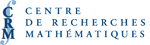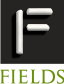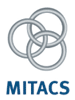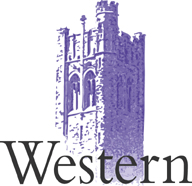# COMMANDITESUniversité Western Ontario
Département de mathématiques
Faculté d'éducation
Faculté des sciences
Recherche Western
Département de mathématiques appliquées

Nous remercions chaleureusement ces commanditaires de leur soutien.

Géométrie non commutative
Org: Masoud Khalkhali (Western)
[PDF]

PAUL BAUM, Penn State University, University Park, PA 16802, USA
Geometric structure in the representation theory of p-adic groups
[PDF]

Let G be a reductive p-adic group. Examples are GL(n,Qp), SL(n,Qp), etc., where Qp is the field of p-adic numbers. The smooth dual of such a group G is (by definition) the set of equivalence classes of irreducible smooth representations of G. A conjecture-due to A.-M. Aubert, P. F. Baum, and R. J. Plymen-states that this smooth dual is a countable disjoint union of complex affine varieties. These varieties are explicitly identified. BC (Baum-Connes) is known to be true for these groups. The new conjecture can be viewed as a much more precise and geometric version of BC. A general principle of NCG appears to play a role here, which is that in many interesting C* algebras there is a naturally arising dense sub-algebra (which is not holomorphically closed) whose purely algebraic periodic cyclic homology is isomorphic (after tensoring with the complex numbers) to the K-theory of the original C* algebra.

KATIA CONSANI, The Johns Hopkins University, 3400 N. Charles St., Baltimore, MD 21218, USA
Motives and Noncommutative Geometry
[PDF]

Since A. Grothendieck proposed the first definition of a "motif", in the early '60s, this notion has undergone an interesting development and enrichment. Very recently, some applications of a new theory of noncommutative motives to number-theory and quantum field theory have being found or about to be developed, with the support of techniques supplied by Noncommutative Geometry and Operator Algebras. This talk will overview the central topic of motives in algebraic geometry and will outline some of the recent applications of the new notion of "endomotive".

GEORGE ELLIOTT, University of Toronto, Toronto, Ontario M5S 2E4
A canonical form for the Pimsner-Voiculescu embedding
[PDF]

The well-known embedding by Pimsner and Voiculescu of the irrational rotation C*-algebra in an AF algebra is very concrete, but is not quite canonical, as the construction requires making infinitely many choices. In particular, it is not clear whether any automorphisms other than inner ones extend to the AF algebra.

Zhuang Niu and I have found a construction which seems much more natural-it is at least much simpler than the original one-, and for which some automorphisms can be seen to extend. The only difficulty is that we have not yet succeeded in showing that the larger algebra is AF.

HEATH EMERSON, University of Victoria
Equivariant correspondences and applications
[PDF]

We describe the Kasparov group KKG (X,Y) in terms of a group KKgeomG(X,Y) of equivalence classes of correspondences from X to Y. Here G is an infinite discrete group and X is a proper G-space and we assume that both X and Y are manifolds. The description requires some discussion of equivariant K-theory groups and in particular when they can be described in terms of equivariant vector bundles. The proof that the geometric and analytic groups coincide is by Kasparov duality.

SASHA GOROKHOVSKY, University of Colorado, Boulder, CO 80309-0395, USA
Algebraic index theorem for Fourier integral operators
[PDF]

In their work on integral geometry, V. Guillemin and S. Sternberg introduced certain classes of Fourier integral operators which form an algebra. We describe the parallel notion in the framework of deformation quantization. We then proceed to establish the algebraic index theorem in this context.

This is a report on work in progress, joint with P. Bressler, R. Nest and B. Tsygan.

LI GUO, Rutgers University at Newark, Newark, New Jersey 07102
Differential Algebraic Birkhoff Decomposition and the renormalization of multiple zeta values
[PDF]

In the Hopf algebra approach of Connes and Kreimer on renormalization of quantum field theory, the renormalization process is viewed as a special case of their Algebraic Birkhoff Decomposition. We give a differential algebra variation of this decomposition and apply this to the study of multiple zeta values.

PIOTR HAJAC, IMPAN, Sniadeckich 8, Warsaw, 00-956 Poland
Yetter-Drinfeld Structures Revisited
[PDF]

At this moment, I can only provide the title of my talk.

JERRY KAMINKER, IUPUI and UC Davis
Matrix integrals and von Neumann algebras of compact groups
[PDF]

We will discuss matrix integrals on the von Neumann algebra of a compact Lie group. The integrals of certain functions have expansions with terms labeled by Riemann surfaces, S. The coefficients are essentially the volumes of the moduli spaces, hom( p1 (S),G )/G. We will also consider how one could use these methods to obtain certain intersection numbers on these moduli spaces.

This is joint work with Motohico Mulase.

MARCELO LACA, University of Victoria, Victoria, BC V8W 3P4
Hecke algebras from groups acting on trees
[PDF]

We show that the Hecke algebras and C*-algebras of some Hecke pairs of groups of automorphisms of homogeneous trees are semigroup crossed products, and use this to study their internal structure and their K-theory.

This is joint work with U. Baumgartner, J. Ramagge, and G. Willis.

HANFENG LI, SUNY at Buffalo, Buffalo, NY 14260, USA
Smooth algebras
[PDF]

Smooth subalgebras of C*-algebras are usually introduced in an ad hoc way. I will show that in certain situations, the specific smooth algebras are the smallest smooth algebras containing the given elements, therefore are determined by the given elements in a natural way.

SNIGDHAYAN MAHANTA, University of Toronto, 40 St. George Street, Room 6290, Toronto, ON M5S 2E4
DG categories in noncommutative geometry
[PDF]

The role of DG (differential graded) categories in noncommutative geometry will be discussed. A notion of noncommutative motives due to Kontsevich will be introduced in this setting. It can be regarded as an algebraic analogue of the analytic endomotives studied by Connes-Consani-Marcolli. Within this framework a special class of Calabi-Yau ones will be introduced and studied.

JOHN PHILLIPS, University of Victoria, Victoria, BC V8W 3P4, Canada
APS Boundary Conditions, KK-Theory and Cuntz-Krieger Systems
[PDF]

We investigate an extension of ideas of Atiyah-Patodi-Singer (APS) to a noncommutative geometry setting. We use a mapping cone construction to turn odd index pairings into even index pairings with APS boundary conditions in the setting of KK-theory. We find that graph C*-algebras provide a natural class of examples for our construction. Moreover, the index pairings coming from APS boundary conditions yield K-theoretic information about these graph C*-algebras.

RAPHAEL PONGE, Toronto
Noncommutative geometry and lower dimensional volumes in Riemannian geometry
[PDF]

In this talk I shall explain how to define "lower dimensional" volumes of any compact Riemannian manifold as the integrals of local Riemannian invariants. For instance a sense can be given to the area and the length of such a manifold in any dimension. The reasoning is motivated by an idea of Connes and involves in an essential way noncommutative geometry and the analysis of Dirac operators on spin manifolds. However, the ultimate definitions of the lower dimensional volumes don't involve noncommutative geometry or spin structures at all.

JOHN ROE, Penn State University
The analytic surgery sequence and eta invariants
[PDF]

I will discuss joint work with Nigel Higson and Jerry Kaminker about a C*-algebraic counterpart to the surgery exact sequence, and its relationship to the results of Keswani, Mathai, etc., about homotopy invariance and vanishing properties of relative eta invariants.

CLAUDE SCHOCHET, Wayne State University, Detroit, MI 48202, US
C*-algebras, gauge groups, and rational homotopy
[PDF]

Let z be a principal PUn-bundle with associated n-dimensional complex matrix bundle over a compact metric space X and let Az denote the unital C*-algebra of sections of this bundle. We determine the rational H-homotopy type of UAz, the group of unitaries of Az. The answer turns out to be independent of the bundle z and depends only upon the rational cohomology of X. We prove analogous results for the gauge group and the projective gauge group of a principal bundle over X with structure group a connected topological group.

This is joint work with N. C. Phillips and John Klein.

XIANG TANG, Washington University
Characteristic classes for trivialized flat Un bundle
[PDF]

In this talk we will describe a way to define characteristic classes for trivialized flat Un bundle using Hopf-cyclic theory. It turns out that many of these classes extend to K-theory of certain C*-algebras. This allows one to define a set of characteristic numbers, generalizing the relative eta invariant, which are homotopy invariant.

This is a joint work with Jerry Kaminker.